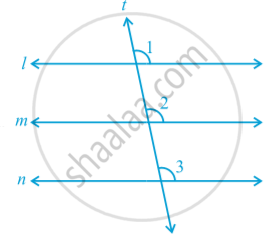# Lines Parallel to the Same Line

#### notes

If two lines are parallel to the same line, will they be parallel to each other in following fig.Line m || line l and line n || line l.
Let us draw a line t transversal for the lines, l, m and n. It is given that line m || line l and line n || line l.
Therefore, ∠ 1 = ∠ 2 and ∠ 1 = ∠ 3
(Corresponding angles axiom)
So, ∠ 2 = ∠ 3
But ∠ 2 and ∠ 3 are corresponding angles and they are equal.
Therefore, you can say that
Line m || Line n
(Converse of corresponding angles axiom)

#### theorem

Theorem:  Lines which are parallel to the same line are parallel to each other.

Note : The property above can be extended to more than two lines also.
Now, let us solve some examples related to parallel lines.

If you would like to contribute notes or other learning material, please submit them using the button below.

### Shaalaa.com

Theorem: Lines which are parallel to the same line are parallel to each other. [00:09:59]
S
0%